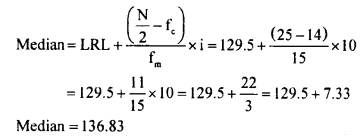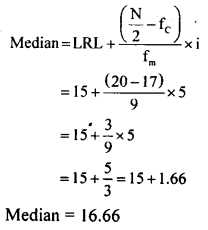# KSEEB Solutions for Class 8 Maths Chapter 13 Statistics Ex 13.3

In this chapter, we provide KSEEB SSLC Class 8 Maths Chapter 13 Statistics Ex 13.3 for English medium students, Which will very helpful for every student in their exams. Students can download the latest KSEEB SSLC Class 8 Maths Chapter 13 Statistics Ex 13.3 pdf, free KSEEB SSLC Class 8 Maths Chapter 13 Statistics Ex 13.3 pdf download. Now you will get step by step solution to each question.

### Karnataka State Syllabus Class 8 Maths Chapter 13 Statistics Ex 13.3

Question 1.
Runs scored by 10 batsmen in a one-day cricket match are given. Find the average run scored. 23, 54, 08, 94, 60, 18, 29, 44, 05, 86
Σ x = 23 + 54 + 08 + 94 + 60 + 18 + 29 + 44 + 05 + 86 = 421
N = 10
Average = Mean =$\overline{X}=\frac{\Sigma x}{N}=\frac{421}{10}=42.1$

Question 2.
Find the mean weight form the following table:

Mean = ΣfXN=47315=31.53

Question 3.
Calculate the mean for the following frequency distribution.

Mean = ΣfxN=171040=42.75

Question 4.
Calculate the mean for the following frequency distribution.

Mean = X¯¯¯¯=ΣfXN=114540=28.625

Question 5.
Find the median of the data 15,22, 9,20, 6,18,11,25,14.
6, 9, 11, 14,(15), 18, 20, 22,25 (Ascending order)
N = 9,$\frac{N+1}{2}+\frac{9+1}{2}=\frac{10}{2}=5^{\text { th }}$
Median = 15.

Question 6.
Find the median of the data 22,28,34, 49, 44, 57,18,10,33, 41, 66, 59.
10, 18, 22, 28, 33, 34, 41, 44, 49, 57, 59, 66 (Ascending order)
N = 10
∴ Median =$\frac{34+41}{2}=\frac{75}{2}=37.5$

Question 7.
Find the median for the following frequency distribution table.

N = 50
N2=5025/2=25
∴ Median class is 130 – 139
LRL = 129.5
Fc = 14
Fm = 15
i = 10Question 8.
Find the median for the following frequency distribution table.

N = 40
N2=402=20
∴ Median class is 15 – 20
LRL = 15
Fc = 17
Fm = 9
i = 5Question 9.
Find the mode for the following data.
(i) 4,3,1,5,3, 7, 9,6 Answer: Mode = 3
(ii) 22,36,18,22,20,34,22, 42, 46,42
Mode = 22

Question 10.
Find the mode for the following data

Mode = 20 (It has the highest frequency)

All Chapter KSEEB Solutions For Class 8 maths

—————————————————————————–

All Subject KSEEB Solutions For Class 8

*************************************************

I think you got complete solutions for this chapter. If You have any queries regarding this chapter, please comment on the below section our subject teacher will answer you. We tried our best to give complete solutions so you got good marks in your exam.

If these solutions have helped you, you can also share kseebsolutionsfor.com to your friends.

Best of Luck!!